10 Systems Simulation

10.1 What it Simulation

10.1.1 Introductory Remark

All models of Operation Research/Management Science can be divided into three groups:

a) Analytical Models

System is represented by means of a system of functions. Examples: Linear Programming, Nonlinear Programming, Transportation models. Markoff systems, Waiting lines models.

b) Topological Models

System is represented using graphs, schemes, diagrams. Examples: CPM, PERT, Maximum flow in network.

c) Simulation Models, Simulations

Systems are modelled using artificial intelligence: computers, processors. Simulation models are excellent means for describing dynamic and stochastic properties of a system. We differentiate

• dynamic systems: elements are moving in space and time
• stochastic system: relations among elements are random and can be described by means of random values and corresponding random distribution function
Simulation techniques are used to describe elements and relations by means of artificial intelligence. Simulation models are used in practice to describe the behaviour of systems in which elements are moving in space and time in a random motion. To describe such motion by means of analytical approach is very difficult.

Simple simulations can be carried out by means of standard software. The more complicated problems must be programmed the more specialised software must be used. Modern program languages enable us construct more simple simulation models using basic program means. Simple simulation models can also be created in spreadsheets.

Simulation models often deal with random numbers and random functions and this is why we use all the principles of the statistical approach.

Computer system users, administrators, and designers usually have a goal of highest performance at lowest cost. Modelling and simulation of system design trade off is good preparation for design and engineering decisions in real world jobs. Simulation is also extensively used to verify the correctness of designs. Most if not all digital integrated circuits manufactured today are first extensively simulated before they are manufactured to identify and correct design errors. Simulation early in the design cycle is important because the cost to repair mistakes increases dramatically the later in the product life cycle that the error is detected.

Another important application of simulation is in developing "virtual environments" , e.g., for training.

System Simulation is the mimicking of the operation of a real system, such as the day-to-day operation of a bank, or the value of a stock portfolio over a time period, or the running of an assembly line in a factory, or the staff assignment of a hospital or a security company, in a computer. Instead of building extensive mathematical models by experts, the readily available simulation software has made it possible to model and analyse the operation of a real system by non-experts, who are managers but not programmers.

A simulation is the execution of a model, represented by a computer program that gives information about the system being investigated. The simulation approach of analysing a model is opposed to the analytical approach, where the method of analysing the system is purely theoretical. As this approach is more reliable, the simulation approach gives more flexibility and convenience. The activities of the model consist of events, which are activated at certain points in time and space and in this way affect the overall state of the system. The points in time that an event is activated can be both deterministic or random. Events exist autonomously and they are discrete so between the execution of two events nothing happens.

The special simulation languages were developed for a process-based approach of writing a simulation program. With this approach, the components of the program consist of entities, which combine several related events into one process.

Newly developed simulation technologies allow senior management to safely play out "what if" scenarios in artificial worlds. For example, in a consumer retail environment it can be used to find out how the roles of consumers and employees can be simulated to achieve peak performance.

10.2 Background to the Simulation Techniques

10.2.1 Computer Simulation

Simulation in general is to pretend that user deals with a real thing while really working with an imitation.

Different technical means can be used to make an imitation:

1. In operations research the imitation is made by an analytic model (in general a system of functions - equations and/or inequalities) which simulate reality.
2. A flight simulator on a PC is also a computer model of some aspects of the flight: it shows on the screen the controls and what the "pilot" (the youngster who operates it) is supposed to see from the "cockpit" (his armchair).
3. Computer simulation. System is described by SW and communication between user-computer is supported by multimedia. Simulations may be performed manually. Most often, however, the system model is written either as a computer program or as some kind of input into simulator software.

10.2.2 The Benefit of Simulations

In many cases, it is very costly, dangerous and often impossible to make experiments with real systems. Provided that models are adequate descriptions of reality (they are valid), experimenting with them can save money, suffering and even time. To fly a simulator is safer and cheaper than the real airplane. For precisely this reason, models are used in industry commerce and military:

Modelling complex dynamic systems theoretically need too many simplifications and the emerging models may not be therefore valid. Simulation does not require that many simplifying assumptions, making it the only tool even in absence of randomness. Simulation can easily describe systems which change with time and space and involve randomness

10.2.3 Types of Simulations

Simulations are classified into two groups:

1. Discrete event

Between two consecutive events, nothing happens. When the number of events are finite, we call the simulation "discrete event." For example the car line: system of arrivals to a crossroads.

2. Continuous

In some systems the state changes all the time, not just at the time of some discrete events. For example, the water level in a reservoir with given in and outflows may change all the time. In such cases "continuous simulation" is more appropriate, although discrete event simulation can serve as an approximation.

10.2.4 Most Frequent Simulation Terminology

1. State: A variable characterising an attribute in the system such as level of stock in inventory or number of jobs waiting for processing.
2. Event: An occurrence at a point in time which may change the state of the system, such as arrival of a customer or start of work on a job.
3. Entity: An object which passes through the system, such as cars in an intersection or orders in a factory. Often an event (e.g., arrival) is associated with an entity (e.g., customer).
4.Queue: A queue is not only a physical queue of people, it can also be a task list, a buffer of finished goods waiting for transportation or any place where entities are waiting for something to happen for any reason.
5. Creating: Creating is causing an arrival of a new entity to the system at some point in time.
6. Scheduling: Scheduling is to assign a new future event to an existing entity.
7. Random variable: A random variable is a quantity which is uncertain, such as interarrival time between two incoming flights or number of defective parts in a shipment.
8. Random variate: A random variate is an artificially generated random variable.
9. Distribution: A distribution is the mathematical law which governs the probabilistic features of a random variable.

10.2.5 A Note About Simulation Software

The vast amount of simulation software available can be overwhelming for the new users. The following are a sample of software in the market today:
ACSL, APROS, ARTIFEX, Arena, AutoMod, C++SIM, CSIM, Call\$im, FluidFlow, GPSS, Gepasi, JavSim, MJX, MedModel, Mesquite, Multiverse, NETWORK, OPNET Modeler, POSES++, Simulat8, Powersim, QUEST, REAL, SHIFT, SIMPLE++, SIMSCRIPT, SLAM, SMPL, SimBank, SimPlusPlus, TIERRA, Witness, and Javasim.

There are several things that make an ideal simulation package. Some are properties of the package, such as support, reactivity to bug notification, interface, etc. Some are properties of the user, such as their needs, their level of expertise, etc. For these reasons asking which package is best is a sudden failure of judgment.

The main question is: What are the important aspects to look for in a package? The answer depends on specific applications. However some general criteria are:

Input facilities, Processing which allows some programming, Optimisation capability, Output facilities, Environment including training and support services, Input-output statistical data analysis capability, and certainly the Cost factor.

10.3 Statistics and Probability for Simulation

Each simulation experiment is a statistical experiment. Statistics and probability are widely used in simulation modelling. Following base knowledge of statistics and probability must reader know:

10.3.1 Random Numbers Generators

The base of each simulation model which describes random behaviour is the random number x .

Random numbers x1, x2, x3, … are real numbers satisfying all three following conditions:

1) xj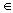(0, 1) .
2) The set of random numbers { xj } is statistic independent.
3) The set of random numbers { xj } has rectangular (steady) probability distribution on interval (0, 1).

Random numbers are created in practice as follows:

• by means of special hardware
• they are presented in tables of random numbers
• as so called pseudo random numbers (PRN), by means of special software (using arithmetic formulas)

•

The procedure which creates random numbers is called generator of random numbers. The diagram below shows the command which creates a random number.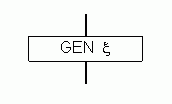10.3.2 Transformation of random numbers to random values

Random numbers can be easily transformed to random value with assigned distribution.
a) Transformation of random number xifrom interval (0, 1) on interval (a, b) is made by formula

h = a + (b – a) x .
Random value h has rectangular distribution on interval (a, b).

b) Transformation of random number xifrom interval (0, 1) to value t with a probability density y = f(x):

1) Probability density f(x) is transformed to cumulative distribution function F(x)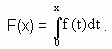2) The solution of the equation
xi = F(ti)

e.g.

ti = F-1(xi)

gives the required random value ti with probability density f(x).

10.3.3 Example

Suppose the cumulative distribution function of interest can be given in a formula which can be graphically replaced by an equivalent algebraic relationship - for example by the exponential probability density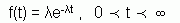1) The cumulative distribution function of the exponential distribution is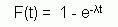2) Now set a random xi equal to the cumulative distribution function and solve for xi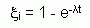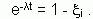10.3.4 Examples

Let’s consider random numbers x1 = 0,2 ; x2 = 0,12 ; x3 = 0,81.

a) Create three random values with rectangular distribution on interval (5,7) using the given random numbers.

b) Generate the random value t with probability distribution f(x) = 4t3 .

a) According to formula h = a + (b – a) x we have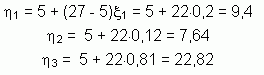b) A random value is expressed by density function and this is why we first determine its cumulative distribution functionNow we solve the equalition x = t4 . For example, use x1 = 0,2. Then 0,2 = t4 and thus t1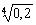= 0,66

10.3.5 Computer Generating Random Numbers and Random Values

Computer programs that generate "random" numbers use an algorithm. That means if you know the algorithm and the seed values you can predict what numbers will result. Because you can predict the numbers they are not truly random - they are pseudorandom. For statistical purposes "good" pseudorandom numbers generators are good enough. Among best known generators behove RANECU (Fortran), Shuffling Routine (Visual Basic), Inverse Gaussian Generator (Easy to programme, user can programme it by himself).

10.4 System Design of Simulation Model

Discrete event systems abound in real world applications. Examples include traffic systems, flexible manufacturing systems, computer-communications systems, production lines, coherent lifetime systems, and flow networks. Most of these systems can be modelled in terms of discrete events whose occurrence causes the system to change from one state to another. In designing, analysing and operating such complex systems, one is interested not only in performance evaluation but also in sensitivity analysis and optimisation.

A typical stochastic system has a large number of control parameters that can have a significant impact on the performance of the system. To establish a basic knowledge of the behaviour of a system under variation of input parameter values and to estimate the relative importance of the input parameters, sensitivity analysis applies small changes to the nominal values of input parameters.

Sensitivity analysis is concerned with evaluating of performance measures with respect to parameters of interest. It provides guidance for design and operational decisions and plays a pivotal role in identifying the most significant system parameters, as well as bottleneck subsystems.

System design of a simulation model can be divided into three distinct processes:
1. Descriptive Analysis. Includes: Problem Identification, Problem Formulation, Data Collection and Analysis, Computer Simulation Model Development, Validation, Verification and Calibration, and finally Performance Evaluation.
2. Prescriptive Analysis: Optimisation or Goal Seeking.
3. Post-prescriptive Analysis: Sensitivity, and What-If Analysis.

10.4.1 Problem Formulation

Identify controllable and uncontrollable inputs. Identify constraints on the decision variable. Define measure of system performance and an objective function. Develop a preliminary model structure to interrelate the inputs and the measure of performance.

10.4.2 Data Collection and Analysis

Regardless of the method used to collect the data, the decision of how much to collect is a trade-off between cost and accuracy.

10.4.3 Simulation Model Development

Acquiring sufficient understanding of the system to develop an appropriate conceptual, logical and then simulation model is one of the most difficult task in simulation analysis.

10.4.4 Model Validation, Verification and Calibration

In general, verification focuses on the internal consistency of a model, while validation is concerned with the correspondence between the model and reality. The term validation is applied to those processes which seek to determine whether or not a simulation is correct with respect to the "real" system. More prosaically, validation is concerned with the question "Are we building the right system?". Verification, on the other hand, seeks to answer the question "Are we building the system right?" Verification checks that the implementation of the simulation model (programme) corresponds to the model. Validation checks that the model corresponds to reality. Calibration checks that the data generated by the simulation matches real (observed) data.

Validation: The process of comparing the model's output with the behaviour of the phenomenon. In other words: comparing model execution to reality (physical or otherwise)

Verification: The process of comparing the computer code with the model to ensure that the code is a correct implementation of the model.

Calibration: The process of parameter estimation for a model. Calibration is a tweaking/tuning of existing parameters and usually does not involve the introduction of new ones, changing the model structure. In the context of optimisation, calibration is an optimisation procedure involved in system identification or during experimental design.

10.4.5 Input and Output Analysis

Discrete-event simulation models typically have stochastic components that mimic the probabilistic nature of the system under consideration. Successful input modelling requires a close match between the input model and the true underlying probabilistic mechanism associated with the system. The input data analysis is to model an element (e.g., arrival process, service times) in a discrete-event simulation given a data set collected on the element of interest. This stage performs intensive error checking on the input data, including external, policy, random and deterministic variables. System simulation experiment is learn about its behaviour. Careful planning, or designing, of simulation experiments is generally a great help, saving time and effort by providing efficient ways to estimate the effects of changes in the model's inputs on its outputs. Statistical experimental-design methods are mostly used in the context of simulation experiments.

10.4.6 Performance Evaluation and What-If Analysis

The what-if' analysis is at the very heart of simulation models.

10.4.7 Sensitivity Estimation

Users must be provided with affordable techniques for sensitivity analysis if they are to understand which relationships are meaningful in complicated models.

10.4.8 Optimisation

Traditional optimisation techniques require gradient estimation. As with sensitivity analysis, the current approach for optimisation requires intensive simulation to construct an approximate surface response function - objective function. Gradient estimation techniques can be used to find maximum or minimum objective function (see section Nonlinear programming).

10.4.9 Report Generating

Report generation is a critical link in the communication process between the model and the end user.

10.5 System Dynamics in Simulations

System Dynamics is the study of system behaviour using the principles of feedback, dynamics and simulation. System dynamics is characterised by:
1. Searching for useful solutions to real problems, especially in social systems (businesses, schools, governments,...) and the environment.
2. Using computer simulation models to understand and improve such systems.
3. Basing the simulation models on mental models, qualitative knowledge and numerical information.
4. Using methods and insights from feedback control engineering and other scientific disciplines to assess and improve the quality of models.
5. Seeking improved ways to translate scientific results into achieved implemented improvement.

10.5.1 Social Simulation

Social scientists have always constructed models of social phenomena. Simulation is an important method for modelling social and economic processes. In particular, it provides a "middle way" between the richness of discursive theorising and rigorous but restrictive mathematical models. There are different types of computer simulation and their application to social scientific problems.

Faster hardware and improved software have made building complex simulations easier. Computer simulation methods can be effective for the development of theories as well as for prediction. For example, macro-economic models have been used to simulate future changes in the economy; and simulations have been used in psychology to study cognitive mechanisms.

The field of social simulation seems to be following an interesting line of inquiry. As a general approach in the field, a "world" is specified with much computational detail. Then the 'world' is simulated (using computers) to reveal some of the 'non-trivial' implications (or "emergent properties") of the "world". When these "non trivial" implications are made known (fed back) in world, apparently it constitutes some "added value".

Artificial Life is an interdisciplinary study enterprise aimed at understanding life-as-it-is and life-as-it-could-be, and at synthesising life-like phenomena in chemical, electronic, software, and other artificial media. Artificial Life redefines the concepts of artificial and natural, blurring the borders between traditional disciplines and providing new media and new insights into the origin and principles of life.

Simulation allows the social scientist to experiment with "artificial societies" and explore the implications of theories in ways not otherwise possible.

10.5.2 Web-based Simulation

Web-based simulation is quickly emerging as an area of significant interest for both simulation researchers and simulation practitioners. This interest in web-based simulation is a natural outgrowth of the proliferation of the World-Wide Web and its attendant technologies, e.g. HTML, HTTP, CGI, etc.,and the surging popularity of, and reliance upon, computer simulation as a problem solving and decision support systems tools.

The appearance of the network-friendly programming language, Java, and of distributed object technologies like the Common Object Request Broker Architecture (CORBA) and the Object Linking and Embedding/Component Object Model (OLE/COM) have had particularly acute effects on the state of simulation practice.

Currently, the researchers in the field of web-based simulation are interested in dealing with topics such as methodologies for web-based model development, collaborative model development over the Internet, Java-based modelling and simulation, distributed modelling and simulation using web technologies, and new applications.

10.5.3 Parallel and Distributed Simulation

The increasing size of the systems and designs requires more efficient simulation strategies to accelerate the simulation process. Parallel and distributed simulation approaches seem to be a promising approach in this direction. Current topics under extensive research are:

1. Synchronisation, scheduling, memory management, randomised and reactive/adaptive algorithms, partitioning and load balancing.
2. Synchronisation in multi-user distributed simulation, virtual reality environments, ICT and interoperability.
3. System modelling for parallel simulation, specification, reuse of models/code, and parallelising existing simulations.
4. Language and implementation issues, models of parallel simulation, execution environments, and libraries.
5. Theoretical and empirical studies, prediction and analysis, cost models, benchmarks, and comparative studies.
6. Computer architectures, R& D, telecommunication networks, manufacturing, dynamic systems, and biological/social systems.
7. Web based distributed simulation such as multimedia and real time applications, fault tolerance, implementation issues, use of Java, and CORBA.

10.5.4 Determination of the interference (warm-up period)

To estimate the long-term performance measure of the system, there are several methods such as Batch Means, Independent Replications and Regenerative Method.

Batch Means is a method of estimating the steady-state characteristic from a single-run simulation. The single run is partitioned into equal size batches large enough for estimates obtained from different batches to be approximately independent. In the method of Batch Means, it is important to ensure that the bias due to initial conditions is removed to achieve at least a covariance stationary waiting time process. An obvious remedy is to run the simulation for a period large enough to remove the effect of the initial bias. During this "warm-up period", no attempt is made to record the output of the simulation. The results are thrown away. At the end of this warm-up period, the waiting time of customers are collected for analysis.

10.6 Optimisation Techniques in simulations

Discrete-event simulation is the primary analysis tool for designing complex systems. However, simulation must be linked with a mathematical optimisation technique to be effectively used for systems design.

Objective function is compiled with outgoing parameters. Imputing values of the model are systematically changed and influence outgoing values of the objective function. Each change of imputing parameter results in one solution. Thus many variants can be derived. Variants are evaluated. Optimum gives that variant which yields maximum/minimum in the objective function.

10.6.1 Objective Function in Optimisation Techniques

Analysing complex discrete event system often requires computer simulation. In these systems, the objective function may not be expressible as an explicit function of the input parameters; rather, it involves some performance measures of the system whose values can be found only by running the simulation model or by observing the actual system. On the other hand, due to the increasingly large size and inherent complexity of most man-made systems, purely analytical means are often insufficient for optimisation. In these cases, one must resort to simulation, with its chief advantage being its generality, and its primary disadvantage being its cost in terms of time and money. Even though, in principle, some systems are analytically tractable, the analytical effort required to evaluate the solution may be so formidable that computer simulation becomes attractive. While the price for computing resources continue to dramatically decrease, one nevertheless can still obtain only a statistical estimate as opposed to an exact solution. For practical purposes, this is quite sufficient.

We consider optimising an objective function with respect to a set of continuous and/or discrete controllable parameters subject to some constraints.

Most used approaches to optimisation in simulations are:

10.6.2 Monte Carlo approach

Performance of the system is measured as an expected value of a random vector. Consider a model with vector parameter p. Let

z(p) = E{Z(x)}

be the steady state expected performance measure, where x is a random vector with known probability density function f(x,p) depends on p, and Z is the performance measure.

In discrete event systems, Monte Carlo simulation is usually needed to estimate z(p) for a given value p = p0. By the law of large numbers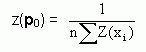converges to the true value, where xi, i = 1, 2,..., n are independent, identically distributed, random vector realisations of x from f(x,p0), and n is the number of independent replications.

Suppose that we seek for optimum value (maximum or minimum) of an random outputting parameter pj. An estimate for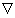p = (p/pj) is a good local measure of the effect of on performance. The sign of the derivative (p/pj) at some point pi immediately gives us the direction in which p should be changed. The magnitude of (p/pj) also provides useful information in an initial design process: If (p/pj) is small, we conclude that z(p) is not very sensitive to changes in inputs, and hence focusing concentration on other parameters may improve performance.

Often sensitivity analysis provides not only a numerical value for the sample derivative, but also an expression which captures the nature of the dependence of a performance measure on the parameter pj. The simplest case arises whenp can be seen to be always positive (or always negative) for any sample path; we may not be able to tell if the value of z(p) is monotonically increasing (or decreasing) in pj. This information in itself is very useful in design and analysis. More generally, the form ofp can reveal structural properties of the model: convexity/concavity.

10.6.4 Perturbation analysis

Impute pj is changed by a "small" amount without actually making this change. Following questions are analysed:

• How does a change in the value of a parameter vary the sample duration related to that parameter?
• How does the change in an individual sample duration reflect itself as a change in a subsequent particular sample realisation?
• What is the relationship between the variation of the sample realisation and its expected value?

• 10.6.5 Score function methods

Using the score function method, the gradient can be estimated simultaneously, at any number of different parameter values, in a single-run simulation. The basic idea is that the gradient of the performance measure function z(p), is expressed as an expectation with respect to the same distribution as the performance measure function itself. Therefore, the sensitivity information can be obtained with little computational (not simulation) cost, while estimating the performance measure. It is well known that the crude form of the Score Function estimator suffers from the problem of linear growth in its variance as the simulation run increases. However, in the steady state simulation the variance can be controlled by run length.

Furthermore, information about the variance may be incorporated into the simulation algorithm. A recent flurry of activity has attempted to improve the accuracy of the Score Function estimates. Under regenerative conditions, the estimator can easily be modified to alleviate this problem, yet the magnitude of the variance may be large for queueing systems with heavy traffic intensity. The heuristic idea is to treat each queue separately, which synchronously assumes that individual components have "local" regenerative cycles. This approach is promising since the estimator remains unbiased and efficient while the global regenerative cycle is very long.

10.6.6 Harmonic analysis

Another strategy for estimating the gradient simulation is based on the frequency domain method, which differs from the time domain experiments in that the input parameters are deterministically varied in sinusoidal patterns during the simulation run, as opposed to being kept fixed as in the time domain runs. The range of possible values for each input factor should be identified. Then the values of each input factor within its defined range should be changed during a run. In time series analysis, t is the time index. In simulation, however, t is not necessarily the simulation clock time. Rather, t is a variable of the model which keeps track of certain statistics during each run. For example, to generate the inter-arrival times in a queueing simulation, t might be the variable that counts customer arrivals.

Frequency domain simulation experiments identify the significant terms of the polynomial that approximates the relationship between the simulation output and the inputs. Clearly, the number of simulation runs required to identify the important terms by this approach is much smaller than those of the competing alternatives, and the difference becomes even more conspicuous as the number of parameters increases.

10.7 What-If and Goal-seeking Techniques

Discrete event simulation is the primary analysis tool for designing complex systems. Simulation, however, must be linked with a optimisation techniques to be effectively used for systems design. We present several optimisation techniques involving both continuous and discrete controllable input parameters subject to a variety of constraints. The aim is to determine the techniques most promising for a given simulation model.

Many man made systems can be modelled as discrete event systems; examples are computer systems, communication networks, flexible manufacturing systems, production assembly lines, and traffic transportation systems. Discrete event simulations system evolve with the occurrence of discrete events, such as the arrival of a job or the completion of a task, in contrast with continuously variable dynamic processes such as aerospace vehicles, which are primarily governed by differential equations. Owing to the complex dynamics resulting from stochastic interactions of such discrete events over time, the performance analysis and optimisation of discrete events system can be difficult tasks. At the same time, since such systems are becoming more widespread as a result of modern technological advances, it is important to have tools for analysing and optimising the parameters of these systems.

Techniques for searching of optimum of an objective function - in simulation model represented by response surface - are based on algorithms of nonlinear programming. All methods are rather complex and require collaboration of specialists. Following view of methods should reader to orientate oneself in the problem.

10.7.1 Deterministic Search Techniques

A common characteristic of deterministic search techniques is that they are basically borrowed from deterministic optimisation techniques. The deterministic objective function value required in the technique is now replaced with an estimate obtained from simulation. By having a reasonably accurate estimate, one hopes that the technique will perform well.

Deterministic search techniques include heuristic search, complete enumeration, and random search techniques.

10.7.2 Heuristic Search Technique

The heuristic search technique is probably most commonly used in optimising objective functions represented by response surfaces. It is also the least sophisticated scheme mathematically, and it can be thought of as an intuitive and experimental approach. The analyst determines the starting point and stopping rule based on previous experience with the system. After setting the input parameters to levels that appear reasonable, the analyst makes a simulation run with the factors set at those levels and computes the value of the response function. If it appears to be a maximum/minimum to the analyst, the experiment is stopped. Otherwise the analyst changes parameter settings and makes another run. This process continues until the analyst believes that the output has been optimised.

This procedure can turn into a blind search and can expend an inordinate amount of time and computer resources without producing results commensurate with input. The heuristic search can be ineffective and inefficient in the hand of a novice.

10.7.3 Complete Enumeration and Random Techniques

The complete enumeration technique is applicable in discrete space yields the optimal value of the objective function. All impute and outpute factors assume a finite number of values for this technique to be applicable. Then, a complete factorial experiment is run. The analyst can attribute some degree of confidence to the determined optimal point when using this procedure.

Although the complete enumeration technique yields the optimal point, it has a serious drawback. If the number of factors or levels per factor is large, the number of simulation runs required to find the optimal point can be exceedingly large.

The random search technique resembles the complete enumeration technique except that one selects a set of inputs at random. The simulated results based on the set that yields the maximum (minimum) value of the response function is taken to be the optimal point. This procedure reduces the number of simulation runs required to yield an optimal result; however, there is no guarantee that the point found is actually the optimal point. Of course, the more points selected, the more likely the analyst is to achieve the true optimum. Note that the requirement that each factor assume only a finite number of values is not a requirement in this scheme. Replications can be made on the treatment combinations selected, to increase the confidence in the optimal point. Which strategy is better, replicating a few points or looking at a single observation on more points, depends on the problem.

10.7.4 Response Surface Search

Response surface search attempts to fit a polynomial to z(p). If the design space is suitably small, the performance function z(p) may be approximated by a response surface, typically a first order, or perhaps quadratic order in z(p). The response surface method requires running the simulation in a first order experimental design to determine the path of steepest descent (see section Nonlinear Programming). Simulation runs made along this path continue, until one notes no improvement in z(p). The analyst then runs a new first order experimental design around the new "optimal" point reached, and finds a new path of steepest descent. The process continues, until there is a lack of fit in the fitted first order surface. Then, one runs a second order design, and takes the optimum of the fittest second order surface as the estimated optimum.

Although it is desirable for search procedures to be efficient over a wide range of response surfaces, no current procedure can effectively overcome case when surface has more than one local maximum or minimum. An obvious way to find the global optimal would be to evaluate all the local optima. One technique that is used when this unimodality is known to exist, is called the "Las Vegas" technique.

10.7.5 Pattern Search Techniques

Pattern search techniques assume that any successful set of moves used in searching for an approximated optimum is worth repeating. These techniques start with small steps; then, if these are successful, the step size increases. Alternatively, when a sequence of steps fails to improve the objective function, this indicates that shorter steps are appropriate so we may not overlook any promising direction. These techniques start by initially selecting a set of incremental values for each factor. Starting at an initial base point, they check if any incremental changes in the first variable yields an improvement. The resulting improved setting becomes the new intermediate base point. One repeats the process for each of the inputs until one obtains a new setting where the intermediate base points act as the initial base point for the first variable. The technique then moves to the new setting. This procedure is repeated, until further changes cannot be made with the given incremental values. Then, the incremental values are decreased, and the procedure is repeated from the beginning. When the incremental values reach a pre-specified tolerance, the procedure terminates; the most recent factor settings are reported as the solution.

10.7.6 Conjugate Direction Search

The conjugate direction search (also conjugate gradient method) requires no derivative estimation, yet it finds the optimum of an n-dimensional quadratic surface after, at most, n-iterations, where the number of iterations is equal to the dimension of the quadratic surface. The procedure redefines the n dimensions so that a single variable search can be used successively. Single variable procedures can be used whenever dimensions can be treated independently. The optimisation along each dimension leads to the optimisation of the entire surface.

Two directions are defined to be conjugate whenever the cross product terms are all zero. The conjugate direction technique tries to find a set of n dimensions that describes the surface such that each direction is conjugate to all others.

Using the above result, the technique attempts to find two search optima and replace the n-th dimension of the quadratic surface by the direction specified by the two optima. Successively replacing the original dimension yields a new set of n dimensions in which, if the original surface is quadratic, all directions are conjugate to each other and appropriate for n single variable searches. While this search procedure appears to be very simple, we should point out that the selection of appropriate step sizes is most critical. The step size selection is more critical for this search technique because - during axis rotation - the step size does not remain invariant in all dimensions. As the rotation takes place, the best step size changes, and becomes difficult to estimate.

10.7.7 Method of Steepest Ascent

The steepest ascent (descent) technique uses a fundamental result from mathematical analysis that the gradient points in the direction of the maximum increase of a function. This enable us to determine how the initial settings of the parameters should be changed to yield an optimal value of the response variable. The direction of movement is made proportional to the estimated sensitivity of the performance of each variable.

Although quadratic functions are sometimes used, one assumes that performance is linearly related to the change in the controllable variables for small changes. Assume that a good approximation is a linear form. The basis of the linear steepest ascent is that each controllable variable is changed in proportion to the magnitude of its slope. When each controllable variable is changed by a small amount, it is analogous to determining the gradient at a point. For a surface containing n controllable variables, this requires n points around the point of interest. When the problem is not an n-dimensional elliptical surface, the parallel tangent points are extracted from bitangents and inflection points of occluding contours. Parallel tangent points are points on the occluding contour where the tangent is parallel to a given bitangent or the tangent at an inflection point.

10.7.8 Tabu Search Technique

An effective technique to overcome local optimality for discrete optimisation is the Tabu Search technique. It explores the search space by moving from a solution to its best neighbor, even if this results in a deterioration of the performance measure value. This approach increases the likelihood of moving out of local optima. To avoid cycling, solutions that were recently examined are declared tabu for a certain number of iterations. Applying intensification procedures can accentuate the search in a promising region of the solution space. In contrast, diversification can be used to broaden the search to a less explored region. Much remains to be discovered about the range of problems for which the tabu search is best suited.

10.7.9 Simplex Based Techniques

The simplex based technique performs simulation runs first at the vertices of the initial simplex; i.e., a polyhedron in the n-space having n+1 vertices. A subsequent simplex (moving towards the optimum) are formed by three operations performed on the current simplex: reflection, contraction, and expansion. At each stage of the search process, the point with the highest z(p) is replaced with a new point found via reflection through the simplex vertices. Depending on the value of z(p) at this new point, the simplex is either expanded, contracted, or unchanged. The simplex technique starts with a set of n+1 factor settings. These n+1 points are all the same distance from the current point. Moreover, the distance between any two points of these n+1 points is the same. Then, by comparing their response values, the technique eliminates the factor setting with the worst functional value and replaces it with a new better factor setting. The resulting simplex either grows or shrinks; it depends on the response value at the new factor settings. One repeats the procedure until no more improvement can be made by eliminating a point, and the resulting final simplex is small. While this technique will generally performance well for unconstrained problems, it may collapse to a point on a boundary of a feasible region, thereby causing the search to come to a premature halt. This technique is effective if the response surface is generally bowl- shaped even with some local optimal points.

10.7.10 Probabilistic Search Techniques

All probabilistic search techniques select trial points governed by a scan distribution, which is the main source of randomness. These search techniques include random search, pure adaptive techniques, simulated annealing, and genetic methods.

10.7.11 Techniques of Generation of the Initial Solution

When performing search techniques in general, and simulated genetic techniques specifically, the question of how to generate the initial solution arises. Theoretically, it should not matter, but in practice this may depend on the problem. In some cases, a pure random solution systematically produces better final results. On the other hand, a good initial solution may lead to lower overall run times. This can be important, for example, in cases where each iteration takes a relatively long time; therefore, one has to use some clever termination rule. Simulation time is a crucial bottleneck in an optimisation process. In many cases, a simulation is run several times with different initial solutions. Such a technique is most robust, but it requires the maximum number of replications compared with all other techniques. The pattern search technique applied to small problems with no constraints or qualitative input parameters requires fewer replications than the genetic techniques. Genetic techniques can easily handle constraints, and have lower computational complexity.

• can quickly get to the vicinity of the optimal solution because its orientation is global
• uses gradient surface fitting, rather than direct performance response surface fitting through single run gradient estimators
• technique may be implemented while the system is running (online technique)

• A typical optimisation scheme involves two phases:

• search phase
• Iteration phase

• Most results in analytic computational complexity assume that good initial approximations are available, and deal with the iteration phase only. If enough time is spent in the initial search phase, we can reduce the time needed in the iteration phase.

10.8 Goal Seeking Problem

10.8.1 General Problem of Goal seeking

In some situations, the decision maker may not be interested in optimisation but wishes to achieve a certain value for z(p), say z(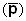). This is the goal seeking problem: given a target output value z(p) of the performance and a parameterised distribution function family, one must find an input value for the parameter which generates such an output.

When is a controllable input, the decision maker may be interested in the goal seeking problem: namely, what change of the input parameter will achieve a desired change in the output value.

Another application of the goal seeking problem arises when we want to adapt a model to satisfy a new equality constraint with some stochastic functions. We may apply the search techniques, but the goal seeking problem can be considered as an interpolation based on a metamodel. In this approach, one generates a response surface function for z(p). Finally, one uses the fitted function to interpolate for the unknown parameter. This approach is tedious, time consuming, and costly; moreover, in a random environment, the fitted model might have unstable coefficients.

The goal seeking problem can be solved as a simulation problem. By this approach, we are able to apply variance reduction techniques used in the simulation literature. Specifically, the solution to the goal-seeking problem is the unique solution of the stochastic equation z(p)- z()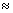0. The problem is to solve this stochastic equation by a suitable experimental design, to ensure convergence and reach required accuracy. It can be performed by random approach, for example by Monte Carlo techniques.

10.8.2 Post Solution Analysis

Stochastic models typically depend upon various uncertain and uncontrollable input parameters that must be estimated from existing data sets. We focus on the statistical question of how input parameter uncertainty propagates through the model into output parameter uncertainty. The sequential stages are descriptive, prescriptive and post prescriptive analysis.

10.8.3 Rare Event Simulation

Large deviations can be used to estimate the probability of rare events, such as buffer overflow, in queueing networks. It is simple enough to be applied to very general traffic models, and sophisticated enough to give insight into complex behaviour.

Simulation has numerous advantages over other approaches to performance and dependability evaluation; most notably, its modelling power and flexibility. For some models, however, a potential problem is the excessive simulation effort (time) required to achieve the desired accuracy. In particular, simulation of models involving rare events, such as those used for the evaluation of communications and highly-dependable systems, is often not feasible using standard techniques. In recent years, there have been significant theoretical and practical advances towards the development of efficient simulation techniques for the evaluation of these systems approaches.

10.9 Contemporary State of Simulations

With the growing incidence of computer modelling and simulation, the scope of simulation domain must be extended to include much more than traditional optimisation techniques. Optimisation techniques for simulation must also account specifically for the randomness inherent in estimating the performance measure and satisfying the constraints of stochastic systems.

General comparisons among different techniques in terms of bias, variance, and computational complexity are not possible. No single technique works effectively and/or efficiently in all cases.

The simplest technique is the random selection of some points in the search region for estimating the performance measure. In this technique, one usually fixes the number of simulation runs and takes the smallest (or largest) estimated performance measure as the optimum. This technique is useful in combination with other techniques to create a multi start technique for global optimisation. The most effective technique to overcome local optimality for discrete optimisation is the Tabu Search technique. In general, the probabilistic search techniques, as a class, offer several advantages over other optimisation techniques based on gradients. In the random search technique, the objective function can be non smooth or even have discontinuities. The search program is simple to implement on a computer, and it often shows good convergence characteristics.

Genetic techniques are most robust and can produce near best solutions for larger problems. The pattern search technique is most suitable for small size problems with no constraint, and it requires fewer iterations than the genetic techniques. The most promising techniques are the stochastic approximation, simultaneous perturbation, and the gradient surface methods.

Response surface methods have a slow convergence rate, which makes them expensive. The gradient surface method combines the advantages of the response surface methods and efficiency of the gradient estimation techniques. Zero points of the successively approximating gradient surface are then taken as the estimates of the optimal solution.

Genetic simulated method is characterised by several attractive features: it is a single run technique and more efficient than response surface methods at each iteration step, it uses the information from all of the data points rather than just the local gradient; it tries to capture the global features of the gradient surface and thereby quickly arrive in the vicinity of the optimal solution, but close to the optimum, they take many iterations to converge to stationary points. Search techniques are therefore more suitable as a second phase. The main interest is to figure out how to allocate the total available computational budget across the successive iterations.